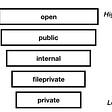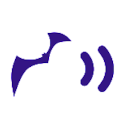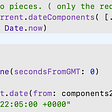# Finding an Affine Transform the Traditional Way with three 2D point correspondences in Swift

1. Populate two 3x3 matrices with the three 2D point pairs.
2. M * A = B, where M = affine matrix, A = “from” points, B = “to” points, all expressed as 3x3 matrices
3. M = B * Inv(A)
4. Find Inv(A), the inverse of matrix A (the “from” points)
5. Create a CGAffineTransform from the 3x3 matrix M
`import CoreGraphicsimport Foundationextension Date {    static func - (lhs: Date, rhs: Date) -> TimeInterval {            return lhs.timeIntervalSinceReferenceDate - rhs.timeIntervalSinceReferenceDate    }}extension CGPoint {    static func - (lhs: CGPoint, rhs: CGPoint) -> CGPoint {        return CGPoint(x: lhs.x - rhs.x, y: lhs.y - rhs.y)    }}extension NumberFormatter {    func string(_ value: CGFloat, digits: Int, failText: String = "[?]") -> String {        minimumFractionDigits = max(0, digits)        maximumFractionDigits = minimumFractionDigits                guard let s = string(from: NSNumber(value: Double(value))) else {            return failText        }                return s    }        func string(_ point: CGPoint, digits: Int = 1, failText: String = "[?]") -> String {        let sx = string(point.x, digits: digits, failText: failText)        let sy = string(point.y, digits: digits, failText: failText)        return "(\(sx), \(sy))"    }        func string(_ vector: CGVector, digits: Int = 1, failText: String = "[?]") -> String {        let sdx = string(vector.dx, digits: digits, failText: failText)        let sdy = string(vector.dy, digits: digits, failText: failText)        return "(\(sdx), \(sdy))"    }        func string(_ transform: CGAffineTransform, rotationDigits: Int = 2, translationDigits: Int = 1, failText: String = "[?]") -> String {        let sa = string(transform.a, digits: rotationDigits)        let sb = string(transform.b, digits: rotationDigits)        let sc = string(transform.c, digits: rotationDigits)        let sd = string(transform.d, digits: rotationDigits)        let stx = string(transform.tx, digits: translationDigits)        let sty = string(transform.ty, digits: translationDigits)        var s = "a:  \(sa)   b: \(sb)   0"        s += "\nc:  \(sc)   d: \(sd)   0"        s += "\ntx: \(stx)   ty: \(sty)   1"        return s    }}/// Indicates are m(row,column)/// |  m11  m12  m13 |/// |  m21  m22  m23 |/// |  m31  m32  m33 |struct Matrix3x3 {    var m11: CGFloat    //row 1    var m12: CGFloat    var m13: CGFloat    var m21: CGFloat    //row 2    var m22: CGFloat    var m23: CGFloat    var m31: CGFloat    //row 3    var m32: CGFloat    var m33: CGFloat        func inverted() -> Matrix3x3? {        let d = determinant()                //TODO pick some realistic near-zero number here        if abs(d) < 0.0000001 {            return nil        }        //transpose matrix first        let t = self.transpose()                //determinants of 2x2 minor matrices        let a11 = t.m22 * t.m33 - t.m32 * t.m23        let a12 = t.m21 * t.m33 - t.m31 * t.m23        let a13 = t.m21 * t.m32 - t.m31 * t.m22                let a21 = t.m12 * t.m33 - t.m32 * t.m13        let a22 = t.m11 * t.m33 - t.m31 * t.m13        let a23 = t.m11 * t.m32 - t.m31 * t.m12                let a31 = t.m12 * t.m23 - t.m22 * t.m13        let a32 = t.m11 * t.m23 - t.m21 * t.m13        let a33 = t.m11 * t.m22 - t.m21 * t.m12                //adjugate (adjoint) matrix: apply + - + ... pattern        let adj = Matrix3x3(            m11: a11, m12: -a12, m13: a13,            m21: -a21, m22: a22, m23: -a23,            m31: a31, m32: -a32, m33: a33)        return adj / d    }        func determinant() -> CGFloat {        m11 * (m22 * m33 - m32 * m23) - m12 * (m21 * m33 - m31 * m23) + m13 * (m21 * m32 - m31 * m22)    }        func transpose() -> Matrix3x3 {        Matrix3x3(m11: m11, m12: m21, m13: m31, m21: m12, m22: m22, m23: m32, m31: m13, m32: m23, m33: m33)    }        /// |  a11  a12  a13 |      |  b11  b12  b13 |    /// |  a21  a22  a23 | *   |  b21  b22  b23 |    /// |  a31  a32  a33 |      |  b31  b32  b33 |    static func * (_ a: Matrix3x3, _ b: Matrix3x3) -> Matrix3x3 {        return Matrix3x3(            m11: a.m11 * b.m11 + a.m12 * b.m21 + a.m13 * b.m31,            m12: a.m11 * b.m12 + a.m12 * b.m22 + a.m13 * b.m32,            m13: a.m11 * b.m13 + a.m12 * b.m23 + a.m13 * b.m33,                        m21: a.m21 * b.m11 + a.m22 * b.m21 + a.m23 * b.m31,            m22: a.m21 * b.m12 + a.m22 * b.m22 + a.m23 * b.m32,            m23: a.m21 * b.m13 + a.m22 * b.m23 + a.m23 * b.m33,                        m31: a.m31 * b.m11 + a.m32 * b.m21 + a.m33 * b.m31,            m32: a.m31 * b.m12 + a.m32 * b.m22 + a.m33 * b.m32,            m33: a.m31 * b.m13 + a.m32 * b.m23 + a.m33 * b.m33)    }        static func / (_ m: Matrix3x3, _ s: CGFloat) -> Matrix3x3 {        Matrix3x3(            m11: m.m11/s, m12: m.m12/s, m13: m.m13/s,            m21: m.m21/s, m22: m.m22/s, m23: m.m23/s,            m31: m.m31/s, m32: m.m32/s, m33: m.m33/s)    }}struct Triangle {    var p1: CGPoint    var p2: CGPoint    var p3: CGPoint        init(p1: CGPoint, p2: CGPoint, p3: CGPoint) {        self.p1 = p1        self.p2 = p2        self.p3 = p3    }        init(x1: CGFloat, y1: CGFloat, x2: CGFloat, y2: CGFloat, x3: CGFloat, y3: CGFloat) {        p1 = CGPoint(x: x1, y: y1)        p2 = CGPoint(x: x2, y: y2)        p3 = CGPoint(x: x3, y: y3)    }        /// | p1.x    p2.x      p3.x |    /// | p1.y    p2.y      p3.y |    /// |   1       1            1    |    func toMatrix() -> Matrix3x3 {        Matrix3x3(m11: p1.x, m12: p2.x, m13: p3.x, m21: p1.y, m22: p2.y, m23: p3.y, m31: 1, m32: 1, m33: 1)    }}func transform(from: Triangle, to: Triangle) -> CGAffineTransform? {    // following example from https://stackoverflow.com/questions/18844000/transfer-coordinates-from-one-triangle-to-another-triangle    // M * A = B    // M = B * Inv(A)    let A = from.toMatrix()        guard let invA = A.inverted() else {        return nil    }        let B = to.toMatrix()    let M = B * invA        // transpose; Apple matrix has translations in bottom row rather than in rightmost column    let CGM = M.transpose()        return CGAffineTransform(a: CGM.m11, b: CGM.m12, c: CGM.m21, d: CGM.m22, tx: CGM.m31, ty: CGM.m32)}func test(from: Triangle, to: Triangle) {    guard let t = transform(from: from, to: to) else {        print("No transform. Perhaps 'from' triangle is colinear and not invertible.")        return    }    let f = NumberFormatter()    print(f.string(t))    let r1 = from.p1.applying(t)    let r2 = from.p2.applying(t)    let r3 = from.p3.applying(t)    let dt1 = to.p1 - r1    let dt2 = to.p2 - r2    let dt3 = to.p3 - r3        print("\(f.string(dt1)) offset from expected for \(f.string(r1))")    print("\(f.string(dt2)) offset from expected for \(f.string(r2))")    print("\(f.string(dt3)) offset from expected for \(f.string(r3))")}func testTransforms() {    print()    print("** Example from SAM workbook  **")    let a = Triangle(p1: CGPoint(x: 1, y: 1), p2: CGPoint(x: 2, y: 3), p3: CGPoint(x: 3, y: 2))    let b = Triangle(p1: CGPoint(x: 3, y: 2), p2: CGPoint(x: 1, y: 5), p3: CGPoint(x: -2, y: 1))    test(from: a, to: b)    print()    print("** Pure Translation **")    let t1 = Triangle(x1: 0, y1: 0, x2: -2, y2: 3, x3: -5, y3: 3)    let t2 = Triangle(x1: 3, y1: -2, x2: 1, y2: 1, x3: -2, y3: 1)    test(from: t1, to: t2)    print()    print("** Rotation and Translation **")    let r1 = Triangle(x1: 0, y1: 0, x2: 1, y2: 0, x3: 0, y3: 1)    let r2 = Triangle(x1: 6, y1: 5, x2: 5, y2: 5, x3: 6, y3: 4)    test(from: r1, to: r2)    print()    print("** Pure Scaling **")    let s1 = Triangle(x1: 0, y1: 0, x2: 1, y2: 0, x3: 0, y3: 1)    let s2 = Triangle(x1: 0, y1: 0, x2: 5, y2: 0, x3: 0, y3: 5)    test(from: s1, to: s2)    print()    print("** Test in which a triangle has colinear points")    let k1 = Triangle(x1: 1, y1: 1, x2: -2, y2: -2, x3: 5, y3: 5)    let k2 = Triangle(x1: -5, y1: 3, x2: 2, y2: 8, x3: -3, y3: 1)    test(from: k1, to: k2)}testTransforms()`

--

--

--

## More from Gary Bartos

Founder of Echobatix, developing assistive technology for the blind. echobatix@gmail.com

Love podcasts or audiobooks? Learn on the go with our new app.

## Useful Swift Extension## SwiftUI Tutorial — How to Read a JSON File## What is PassKit and how to use it?## Solving practical use-cases of Layout using Constraint layout features## Access Control in Swift: A beginner’s guide## How to serialize and deserialize objects on iOS## Set proper height for UITextView which is inside of UIStackView and UITable.headerView## Which Technology to Choose for Your Next Mobile App Development?## Gary Bartos

Founder of Echobatix, developing assistive technology for the blind. echobatix@gmail.com

## Working with Swift Dates## Batch Remove System for GameplayKit Entities## When Apple CEO Tim Cook first announced Swift in 2014, he predicted that it would be the “next…## You shouldn’t migrate to Apple’s iOS if you’re…